# HDL Implementation of a Digital Up-Converter for LTE

This example shows how to design a digital up-converter (DUC) for radio communication applications such as LTE, and generate HDL code with HDL Coder™.

### Introduction

DUCs are widely used in digital communication transmitters to convert baseband signal to Radio Frequency (RF) or Intermediate Frequency (IF) signals. The DUC operation increases the signal's sampling rate and shifts it to a higher frequency to facilitate subsequent processing stages. The DUC presented here performs sample rate conversion using a 4-stage filter chain followed by complex frequency translation. The example starts by designing the DUC with DSP System Toolbox™ functions in floating point. Each stage is then converted to fixed-point, and then used in a Simulink® model which generates synthesizable HDL code. Two test signals are used to demonstrate and verify the DUC operation:

1. A sinusoid modulated onto a 32 MHz IF carrier.

2. An LTE downlink signal with a bandwidth of 1.4 MHz, modulated onto a 32 MHz IF carrier.

The example measures signal quality by down-converting the output of the floating-point and fixed-point DUCs, and compares the two. Finally, FPGA implementation results are presented.

Note: This example uses `DUCTestUtils`, a helper class containing functions for generating stimulus and analyzing the DUC output. See the `DUCTestUtils.m` file for more info.

### DUC Structure

The DUC consists of a interpolating filter chain, a Numerically Controlled Oscillator (NCO), and a mixer. The filter chain consists of a lowpass interpolator, a halfband interpolator, a CIC compensation interpolator (FIR), a CIC interpolator and gain correction.

The overall response of the filter chain is equivalent to that of a single interpolation filter with the same specification, however, splitting the filter into multiple interpolation stages results in a more efficient design which uses fewer hardware resources.

The first lowpass interpolator implements the precise `Fpass` and `Fstop` characteristics of the DUC. The halfband is an intermediate interpolator. Due to the lower sampling rates, the filters near the beginning of the chain can optimize resource use by sharing multipliers. The CIC compensation interpolator improves the spectral response by compensating for the later CIC droop while interpolating by two. The CIC interpolator provides a large interpolation factor, which makes the filter chain reach upsampling requirements. A block diagram of the DUC is shown below.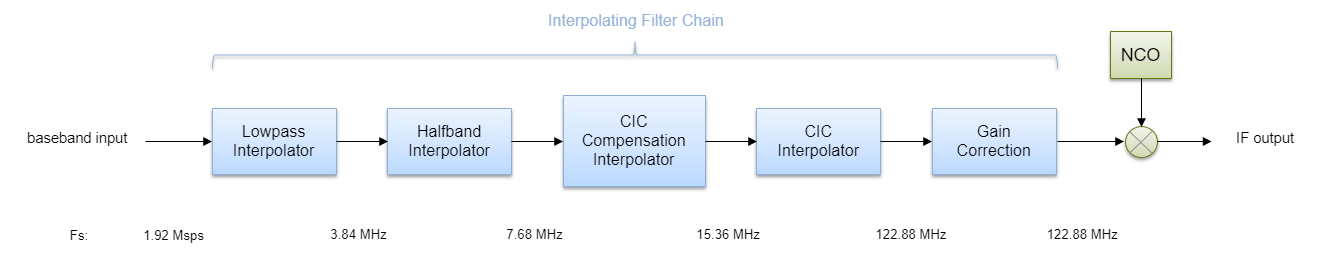The input to the DUC is sampled at 1.92 Msps while the output sample rate is 122.88 Msps. Therefore the overall interpolation factor is 64. 1.92 Msps is the typical sampling rate used by LTE receivers to perform cell search and MIB (Master Information Block) recovery. The DUC filters have therefore been designed to suit this application. The DUC is optimized to run at a clock rate of 122.88 MHz.

### DUC Design

This section explains how to design the DUC using floating-point operations and filter-design functions in MATLAB®. The DUC object allows you to specify several characteristics that define the response of the cascade for the four filters, including passband and stopband frequencies, passband ripple, and stopband attenuation.

DUC Parameters

The desired DUC response is defined by the input sampling rate, carrier frequency, and filter characteristics. Modifying this desired filter response may require changes to the HDL Block Properties of the filter blocks in the Simulink model. HDL Block Properties are discussed later in the example.

```FsIn = 1.92e6; % Sampling rate at input to DUC Fc = 32e6; % Carrier frequency Fpass = 540e3; % Passband frequency, equivalent to 36x15kHz LTE subcarriers Fstop = 700e3; % Stopband frequency Ap = 0.1; % Passband ripple Ast = 60; % Stopband attenuation ```

First Lowpass Interpolator

```lowpassParams.FsIn = FsIn; lowpassParams.InterpolationFactor = 2; lowpassParams.FsOut = FsIn * lowpassParams.InterpolationFactor; lowpassSpec = fdesign.interpolator(lowpassParams.InterpolationFactor,'lowpass',... 'Fp,Fst,Ap,Ast',Fpass,Fstop,Ap,Ast,lowpassParams.FsOut); lowpassFilt = design(lowpassSpec,'SystemObject',true) ```
```lowpassFilt = dsp.FIRInterpolator with properties: NumeratorSource: 'Property' Numerator: [1x69 double] InterpolationFactor: 2 Use get to show all properties ```

Use `fvtool` to display the magnitude response of the lowpass filter without gain correction.

```ducPlots.lowpass = fvtool(lowpassFilt,'Fs',FsIn*2,'Legend','off'); DUCTestUtils.setPlotNameAndTitle('Lowpass Interpolator'); ```Second Halfband Interpolator

``` hbParams.FsIn = lowpassParams.FsOut; hbParams.InterpolationFactor = 2; hbParams.FsOut = lowpassParams.FsOut * hbParams.InterpolationFactor; hbParams.TransitionWidth = hbParams.FsIn - 2 * Fstop; hbParams.StopbandAttenuation = Ast; hbSpec = fdesign.interpolator(hbParams.InterpolationFactor,'halfband',... 'TW,Ast',... hbParams.TransitionWidth,... hbParams.StopbandAttenuation,... hbParams.FsOut); hbFilt = design(hbSpec,'SystemObject',true) ```
```hbFilt = dsp.FIRInterpolator with properties: NumeratorSource: 'Property' Numerator: [1x11 double] InterpolationFactor: 2 Use get to show all properties ```

Visualize the magnitude response of the halfband Interpolation.

```ducFilterChain = dsp.FilterCascade(lowpassFilt,hbFilt); ducPlots.hbFilt = fvtool(lowpassFilt,hbFilt,ducFilterChain,... 'Fs',[FsIn*2,FsIn*4,FsIn*4]); legend(... 'Lowpass Interpolator', ... 'Halfband Interpolator', ... 'Lowpass+Halfband'); DUCTestUtils.setPlotNameAndTitle('Halfband Interpolator'); ```CIC Compensation Interpolator

The magnitude response of the last CIC filter has a significant droop within the passband region, therefore an FIR-based droop compensation filter is used to flatten the passband response. The compensator is configured with the same parameters as the CIC interpolator. This filter also implements interpolation by a factor of two, therefore its bandlimiting characteristics are specified. Specify the filter requirements and then use the `design` function to return a filter System object with those characteristics.

```compParams.FsIn = hbParams.FsOut; compParams.InterpolationFactor = 2; % CIC compensation interpolation factor compParams.FsOut = compParams.FsIn * compParams.InterpolationFactor; % New sampling rate compParams.Fpass = 1/2 * compParams.FsIn + Fpass; % CIC comp passband frequency compParams.Fstop = 1/2 * compParams.FsIn + 1/4 * compParams.FsIn; % CIC comp stopband frequency compParams.Ap = Ap; % Same Ap as overall filter compParams.Ast = Ast; % Same Ast as overall filter ```

The CIC compensation filter structure is also corresponding to later CIC interpolation. So some CIC interpolator parameters are specified here.

```cicParams.InterpolationFactor = 8; % CIC interpolation factor cicParams.DifferentialDelay = 1; % CIC interpolator differential delay cicParams.NumSections = 3; % CIC interpolator number of integrator and comb sections compSpec = fdesign.interpolator(compParams.InterpolationFactor,'ciccomp',... cicParams.DifferentialDelay,... cicParams.NumSections,... cicParams.InterpolationFactor,... 'Fp,Fst,Ap,Ast',... compParams.Fpass,compParams.Fstop,compParams.Ap,compParams.Ast,... compParams.FsOut); compFilt = design(compSpec,'SystemObject',true) ```
```compFilt = dsp.FIRInterpolator with properties: NumeratorSource: 'Property' Numerator: [1x36 double] InterpolationFactor: 2 Use get to show all properties ```

Plot the response of the CIC Compensation Interpolator.

```ducFilterChain = dsp.FilterCascade(lowpassFilt,hbFilt,compFilt); ducPlots.cicComp = fvtool(lowpassFilt,hbFilt,compFilt,ducFilterChain,... 'Fs',[FsIn*2,FsIn*4,FsIn*8,FsIn*8]); legend(... 'Lowpass Interpolator', ... 'Halfband Interpolator', ... 'CIC Comp Interpolator', ... 'Lowpass+Halfband+CIC Comp'); DUCTestUtils.setPlotNameAndTitle('CIC Comp Interpolator'); ```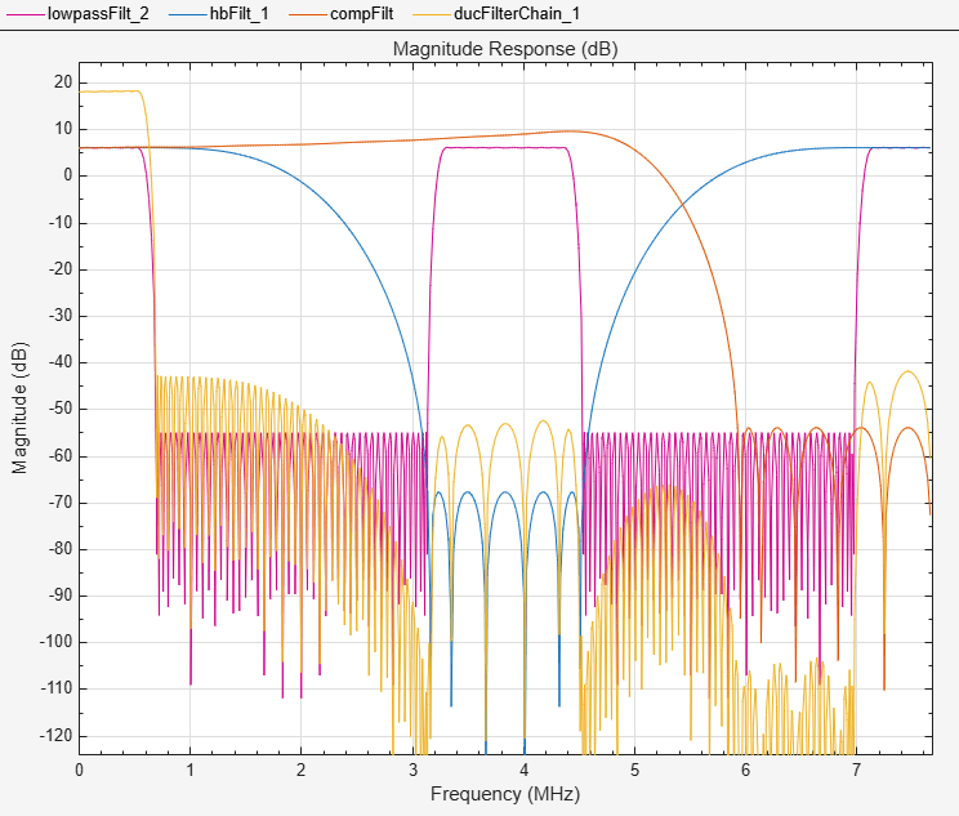CIC Interpolator

The last filter stage is implemented as a CIC interpolator because of its ability to implement a large decimation factor efficiently. The response of a CIC filter is similar to a cascade of moving average filters, however no multiplies or divides are used. As a result, the CIC filter has a large DC gain.

```cicParams.FsIn = compParams.FsOut; cicParams.FsOut = cicParams.FsIn * cicParams.InterpolationFactor; cicFilt = dsp.CICInterpolator(cicParams.InterpolationFactor,... cicParams.DifferentialDelay,cicParams.NumSections) %#ok<*NOPTS> ```
```cicFilt = dsp.CICInterpolator with properties: InterpolationFactor: 8 DifferentialDelay: 1 NumSections: 3 FixedPointDataType: 'Full precision' ```

Visualize the magnitude response of the CIC Interpolation. CIC filters always use fixed-point arithmetic internally, so `fvtool` plots both the quantized and unquantized responses.

```ducFilterChain = dsp.FilterCascade(lowpassFilt,hbFilt,compFilt,cicFilt); ducPlots.cicInter = fvtool(lowpassFilt,hbFilt,compFilt,cicFilt,ducFilterChain,... 'Fs',[FsIn*2,FsIn*4,FsIn*8,FsIn*64,FsIn*64]); legend(... 'First Halfband Interpolator', ... 'Second Halfband Interpolator', ... 'CIC Compensation Interpolator', ... 'CIC Interpolator: Quantized',... 'CIC Interpolator: Reference',... 'Overall Response: Quantized',... 'Overall Response: Reference'); DUCTestUtils.setPlotNameAndTitle('Lowpass + Halfband + CIC Comp + CIC Interpolator'); ```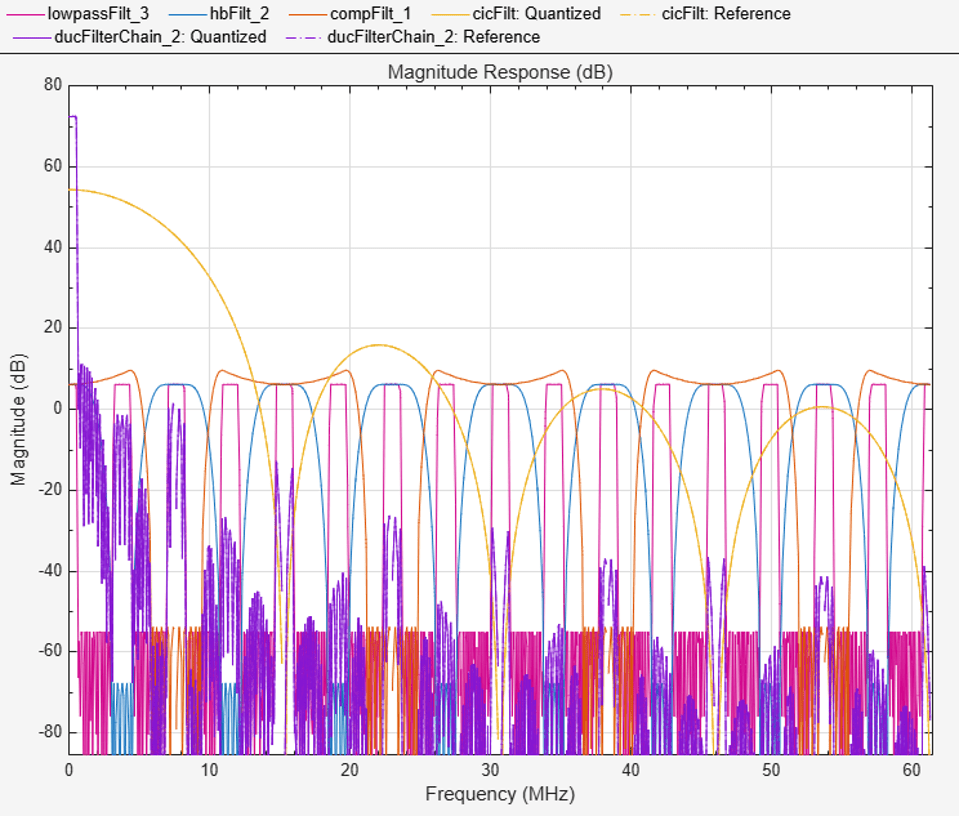For every interpolator, there is a DC gain determined by its interpolation factor. For the CIC interpolator, due to its implementation,it has a larger gain than other filters. Use `gain` to get CIC interpolator's gain. The total gain is a power of two, therefore it can be easily corrected in hardware with a shift operation. For analysis purposes, the gain correction is represented in MATLAB by a one-tap `dsp.FIRFilter` System object. Combine the filter chain and the gain correction filter into a `dsp.FilterCascade` System object.

```cicGain = gain(cicFilt) Gain = lowpassParams.InterpolationFactor * hbParams.InterpolationFactor * compParams.InterpolationFactor * cicParams.InterpolationFactor * cicGain; GainCorr = dsp.FIRFilter('Numerator',1/Gain) ```
```cicGain = 64 GainCorr = dsp.FIRFilter with properties: Structure: 'Direct form' NumeratorSource: 'Property' Numerator: 2.4414e-04 InitialConditions: 0 Use get to show all properties ```

Overall chain response with and without gain correction.

```ducPlots.overallResponse = fvtool(ducFilterChain,dsp.FilterCascade(ducFilterChain,GainCorr),... 'Fs',[FsIn*64,FsIn*64]); DUCTestUtils.setPlotNameAndTitle('Overall DUC Chain Response'); legend(... 'Overall Response: No Gain Correction (Quantized)',... 'Overall Response: No Gain Correction (Reference)',... 'Overall Response: Gain Correction (Quantized)',... 'Overall Response: Gain Correction (Reference)'); ```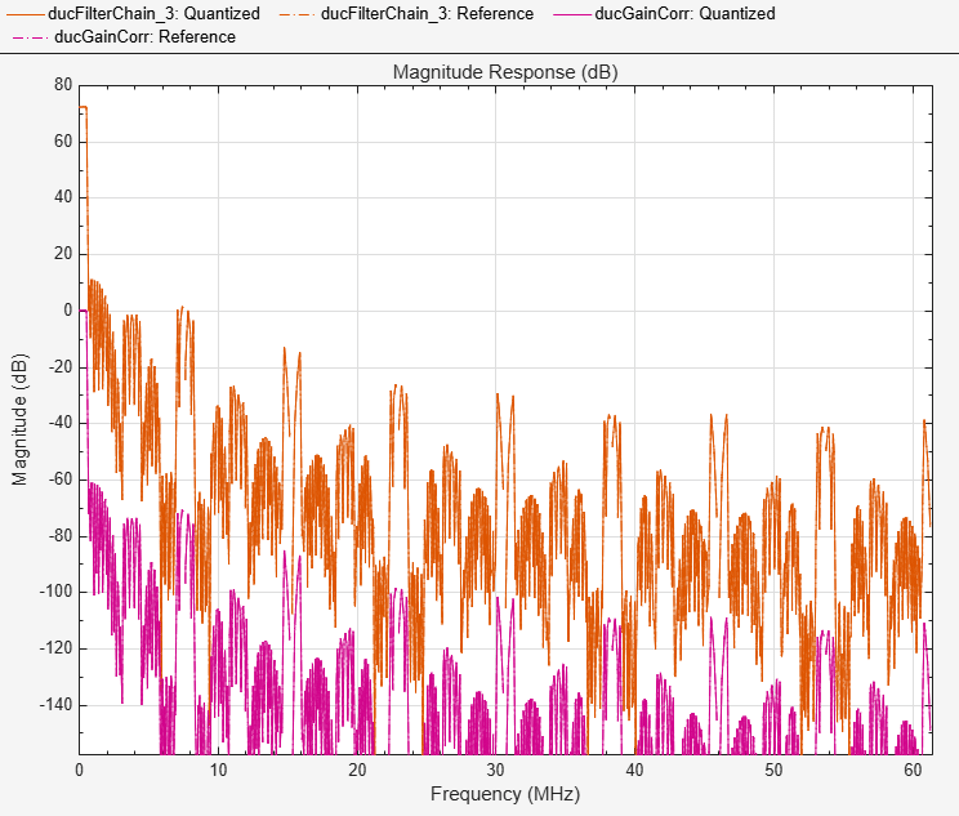### Fixed-Point Conversion

The frequency response of the floating-point DUC filter chain now meets the specification. Next, quantize each filter stage to use fixed-point types and analyze them to confirm that the filter chain still meets the specification.

Filter Quantization

This example uses 16-bit coefficients, which is sufficient to meet the specification. Using fewer than 18 bits for the coefficients minimizes the number of DSP blocks required for an FPGA implementation. The input to the DUC filter chain is 16-bit data with 15 fractional bits. The filter outputs are 18-bit values, which provides extra headroom and precision in the intermediate signals.

```% First Lowpass Interpolator lowpassFilt.FullPrecisionOverride = false; lowpassFilt.CoefficientsDataType = 'Custom'; lowpassFilt.CustomCoefficientsDataType = numerictype([],16,15); lowpassFilt.ProductDataType = 'Full precision'; lowpassFilt.AccumulatorDataType = 'Full precision'; lowpassFilt.OutputDataType = 'Custom'; lowpassFilt.CustomOutputDataType = numerictype([],18,14); % Halfband hbFilt.FullPrecisionOverride = false; hbFilt.CoefficientsDataType = 'Custom'; hbFilt.CustomCoefficientsDataType = numerictype([],16,14); hbFilt.ProductDataType = 'Full precision'; hbFilt.AccumulatorDataType = 'Full precision'; hbFilt.OutputDataType = 'Custom'; hbFilt.CustomOutputDataType = numerictype([],18,14); % CIC Compensation Interpolator compFilt.FullPrecisionOverride = false; compFilt.CoefficientsDataType = 'Custom'; compFilt.CustomCoefficientsDataType = numerictype([],16,14); compFilt.ProductDataType = 'Full precision'; compFilt.AccumulatorDataType = 'Full precision'; compFilt.OutputDataType = 'Custom'; compFilt.CustomOutputDataType = numerictype([],18,14); ```

For the CIC Interpolator, choosing the `Minimum section word lengths` fixed-point data type option automatically optimizes the internal wordlengths based on the output wordlength and other CIC parameters.

```cicFilt.FixedPointDataType = 'Minimum section word lengths'; cicFilt.OutputWordLength = 18; ```

Configure the fixed-point parameters of the gain correction and FIR-based System objects. While not shown explicitly, the object uses the default `RoundingMethod` and `OverflowAction` settings (`Floor` and `Wrap` respectively).

```% CIC Gain Correction GainCorr.FullPrecisionOverride = false; GainCorr.CoefficientsDataType = 'Custom'; GainCorr.CustomCoefficientsDataType = numerictype(fi(GainCorr.Numerator,1,16)); GainCorr.OutputDataType = 'Custom'; GainCorr.CustomOutputDataType = numerictype(1,18,14); ```

Fixed-Point Analysis

Inspect the quantization effects with `fvtool`. The filters can be analyzed individually, or in a cascade. `fvtool` shows the quantized and unquantized (reference) responses overlayed. For example, the effect of quantizing the first FIR filter stage is shown.

```ducPlots.quantizedFIR = fvtool(lowpassFilt,'Fs',lowpassParams.FsIn*2,'arithmetic','fixed'); DUCTestUtils.setPlotNameAndTitle('Quantized Lowpass Interpolator'); legend(... 'Lowpass Interpolator: Quantized', ... 'Lowpass Interpolator: Reference'); ```Redefine the `ducFilterChain` cascade object to include the fixed-point properties of the individual filters. Then use `fvtool` to analyze the entire filter chain and confirm that the quantized DUC still meets the specification.

```ducFilterChain = dsp.FilterCascade(lowpassFilt,hbFilt,compFilt,cicFilt,GainCorr); ducPlots.quantizedDUCResponse = fvtool(ducFilterChain,'Fs',FsIn*64,'Arithmetic','fixed'); DUCTestUtils.setPlotNameAndTitle('Quantized DUC Filter Chain'); legend(... 'DUC filter chain: Quantized', ... 'DUC filter chain: Reference'); ```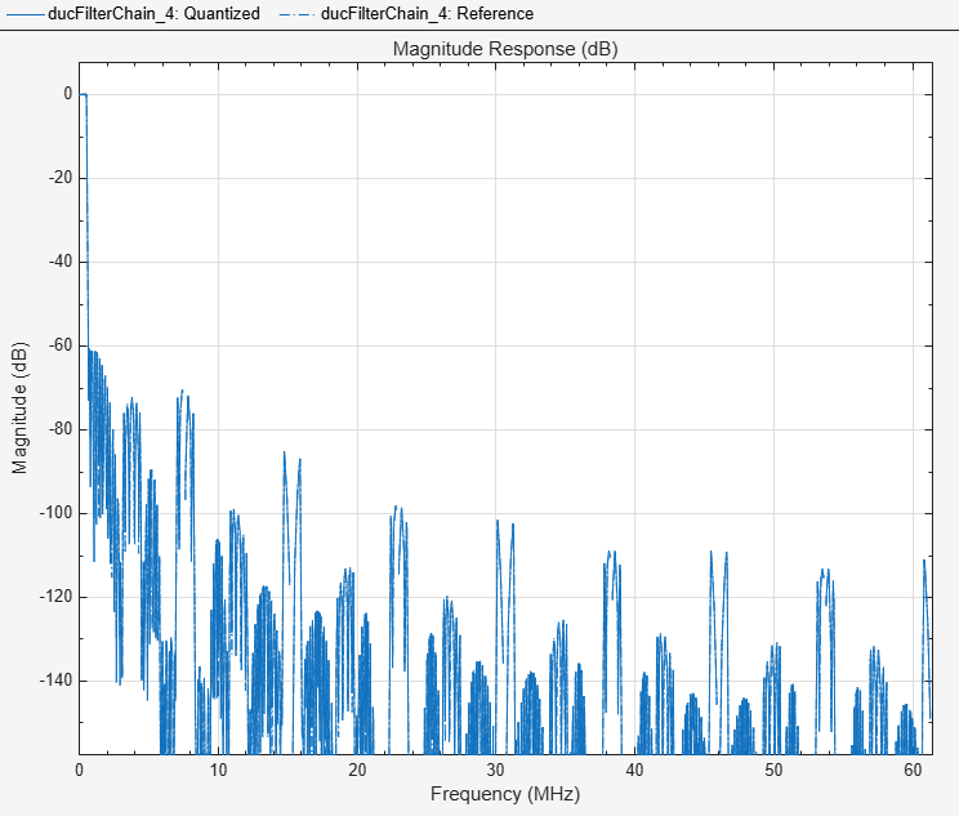The next step in the design flow is to implement the DUC in Simulink using HDL Coder compatible blocks.

Model Configuration

The model relies on variables in the MATLAB workspace to configure the blocks and settings. It uses the filter chain variables already defined. Next, define the Numerically Controlled Oscillator (NCO) parameters, and the input signal.

The input to the DUC comes from `ducIn`. For now, assign a dummy value for `ducIn` so that the model can compute its data types. During testing, `ducIn` provides input data to the model.

```ducIn = 0; %#ok<NASGU> ```

Model Structure

The top level of the DUC Simulink model is shown. The model imports `ducIn` from the MATLAB workspace using a Signal From Workspace block, converts it to 16-bits and then applies it to the DUC. HDL code can be generated from the HDL_DUC subsystem.

```modelName = 'DUCforLTEHDL'; open_system(modelName); set_param(modelName, 'Open','on'); ```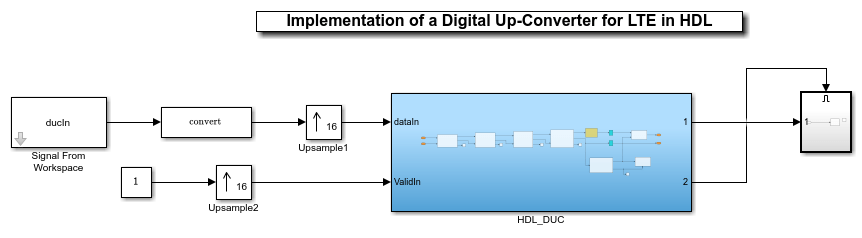The DUC implementation is inside the HDL_DUC subsystem.

```set_param([modelName '/HDL_DUC'],'Open','on'); ```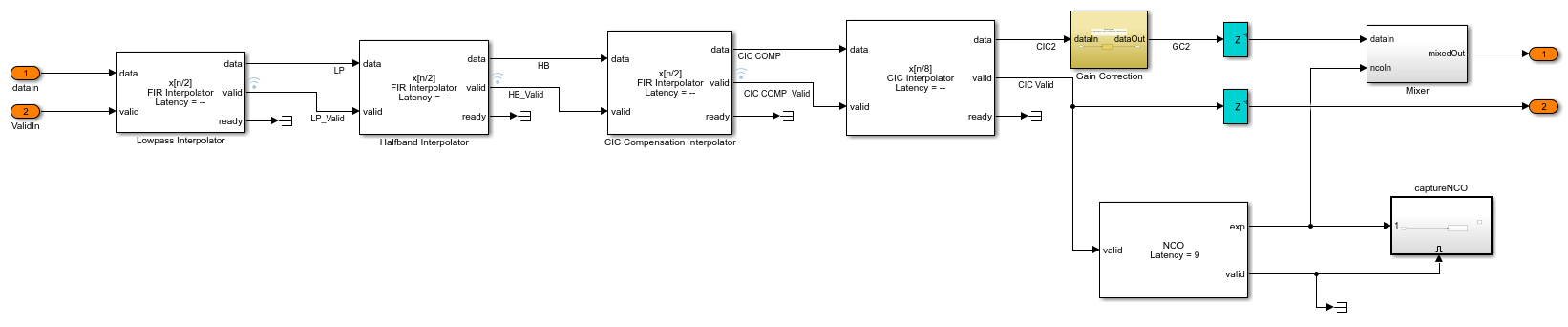Filter Block Parameters

All of the filters are configured to inherit the properties of the corresponding System objects. Each block also has a set of HDL Properties which are used to optimize the resulting HDL code. In particular, the lowpass, Halfband, and CIC Compensation blocks operate at sampling rates which are lower than the clock rate (Fclk) by factors of 32, 16, and 8 respectively. These blocks use serialization techniques (resource sharing) to minimize hardware resource utilization. For example, the input to the Lowpass Interpolation block is sampled at Fclk/32, therefore 32 clock cycles are available to process each input sample. When HDL code is generated for the DUC, HDL Coder lists all of the `SerialPartition` options available for the filter blocks, based on their coefficients. The largest value in the `SerialPartition` vector represents the sharing factor of the filter. In this example, HDL Coder lists the minimum multiplier utilization achieves when the `SerialPartition` of the Lowpass Interpolation block is set to `17`, so that it utilizes 17 clock cycles available to it. For more information see SerialPartition (HDL Coder) and HDL Filter Architectures (HDL Coder).Gain Correction

The gain correction divides the output by 4096, which is equivalent to shifting right by 12 bits. The number of bits at both the input and output of the gain correction is 18-bits, therefore this shift is implemented by simply reinterpreting the data type as shown.

```set_param([modelName '/HDL_DUC/Gain Correction'],'Open','on'); ```NCO Block Parameters

The NCO HDL Optimized block generates a complex phasor at the carrier frequency. This signal goes to a mixer which multiplies it with the output signal. The output of the mixer is sampled at 122.88 Msps. Specify the desired frequency resolution. Calculate the number of accumulator bits required to achieve the desired resolution, and define the number of quantized accumulator bits. The quantized output of the accumulator is used to address the sine lookup table inside the NCO. Also compute the phase increment needed to generate the specified carrier frequency. Phase dither is applied to those accumulator bits which are removed during quantization. These parameters are used to configure the NCO block.

```nco.Fd = 1; nco.AccWL = nextpow2(FsIn*64/nco.Fd) + 1; nco.QuantAccWL = 12; nco.PhaseInc = round((Fc * 2^nco.AccWL)/(FsIn*64)); nco.NumDitherBits = nco.AccWL - nco.QuantAccWL; ```

The NCO block is configured with the parameters defined in the `nco` structure. Both tabs of the block's parameter dialog are shown.### Sinusoid on Carrier Test and Verification

To test the DUC, a 40kHz sinusoid is passed through the DUC and modulated onto the carrier frequency. The modulated signal is then demodulated and resampled at the receiver end. Then measure the Spurious Free Dynamic Range (SFDR) of the resulting tone and the SFDR of the NCO output.

```% Initialize random seed before executing any simulations. rng(0); % Generate a 40kHz test tone ducIn = DUCTestUtils.GenerateTestTone(40e3); % Up convert the test signal with the floating point DUC. ducTx = DUCTestUtils.UpConvert(ducIn,FsIn*64,Fc,ducFilterChain); release(ducFilterChain); % Down convert the output of DUC. ducRx = DUCTestUtils.DownConvert(ducTx,FsIn*64,Fc); % Up convert the test signal by executing the fixed-point Simulink model with the sim function. simOut = sim(modelName); % Downconvert the output of DUC. simTx = simOut.ducOut; simRx = DUCTestUtils.DownConvert(simTx,FsIn*64,Fc); % Measure the SFDR of the NCO, floating point DUC and the fixed-point DUC outputs. results.sfdrNCO = sfdr(real(simOut.ncoOut),FsIn); results.sfdrFloatDUC = sfdr(real(ducRx),FsIn); results.sfdrFixedDUC = sfdr(real(simRx),FsIn); disp('Spurious Free Dynamic Range (SFDR) Measurements'); disp([' Floating point DUC SFDR: ',num2str(results.sfdrFloatDUC) ' dB']); disp([' Fixed-point NCO SFDR: ',num2str(results.sfdrNCO) ' dB']); disp([' Fixed-point DUC SFDR: ',num2str(results.sfdrFixedDUC) ' dB']); fprintf(newline); % Plot the SFDR of the NCO and fixed-point DUC outputs. ducPlots.ncoOutSDFR = figure; sfdr(real(simOut.ncoOut),FsIn); DUCTestUtils.setPlotNameAndTitle(['NCO Out ' get(gca,'Title').String]); ducPlots.ducOutSDFR = figure; sfdr(real(simRx),FsIn); DUCTestUtils.setPlotNameAndTitle(['Fixed-Point DUC Out ' get(gca,'Title').String]); ```
```Spurious Free Dynamic Range (SFDR) Measurements Floating point DUC SFDR: 287.7185 dB Fixed-point NCO SFDR: 86.0718 dB Fixed-point DUC SFDR: 92.8885 dB ```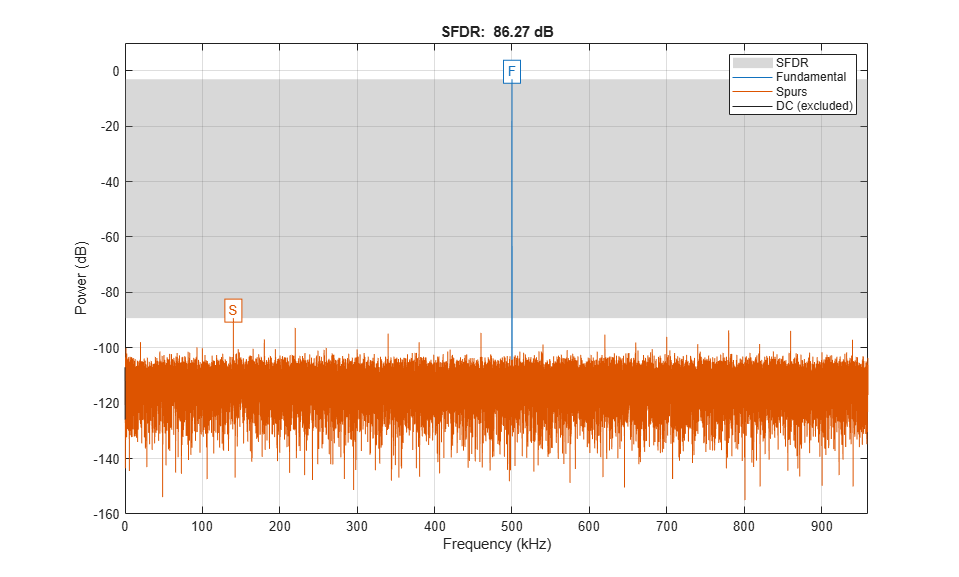### LTE Test and Verification

An LTE test signal is used to perform more rigorous testing of the DUC. LTE Toolbox™ is used to generate a standard compliant LTE waveform. The waveform is up-converted with DUC and then modulated onto the carrier. LTE Toolbox is used to measure the Error Vector Magnitude (EVM) of the resulting signals.

```% Only execute this test if an LTE Toolbox license is present. if license('test','LTE_Toolbox') % Generate a LTE test signal with LTE Toolbox [ducIn, sigInfo] = DUCTestUtils.GenerateLTETestSignal(); % Upconvert with a MATLAB Floating Point Model and modulate onto carrier ducTx = DUCTestUtils.UpConvert(ducIn,FsIn*64,Fc,ducFilterChain); release(ducFilterChain); % Add noise to transmit signal ducTxAddNoise = DUCTestUtils.AddNoise(ducTx); % Downconvert received signal ducRx = DUCTestUtils.DownConvert(ducTxAddNoise,FsIn*64,Fc); % Upconvert using Simulink model simOut = sim(modelName); % Add noise to transmit signal simTx = simOut.ducOut; simTxAddNoise = DUCTestUtils.AddNoise(simTx); % Downconvert received signal simRx = DUCTestUtils.DownConvert(simTxAddNoise,FsIn*64,Fc); results.evmFloat = DUCTestUtils.MeasureEVM(sigInfo,ducRx); results.evmFixed = DUCTestUtils.MeasureEVM(sigInfo,simRx); disp('LTE Error Vector Magnitude (EVM) Measurements'); disp([' Floating point DUC RMS EVM: ' num2str(results.evmFloat.RMS*100,3) '%']); disp([' Floating point DUC Peak EVM: ' num2str(results.evmFloat.Peak*100,3) '%']); disp([' Fixed-point DUC RMS EVM: ' num2str(results.evmFixed.RMS*100,3) '%']); disp([' Fixed-point DUC Peak EVM: ' num2str(results.evmFixed.Peak*100,3) '%']); fprintf(newline); end ```
```LTE Error Vector Magnitude (EVM) Measurements Floating point DUC RMS EVM: 0.782% Floating point DUC Peak EVM: 2.42% Fixed-point DUC RMS EVM: 0.755% Fixed-point DUC Peak EVM: 2.76% ```

### HDL Code Generation and FPGA Implementation

To generate the HDL code for this example you must have an HDL Coder™ license. Use the `makehdl` and `makehdltb` commands to generate HDL code and an HDL testbench for the HDL_DUC subsystem. The DUC was synthesized on a Xilinx® Zynq®-7000 ZC706 evaluation board. The post place and route resource utilization results are shown in the table. The design met timing with a clock frequency of 158 MHz.

```T = table(... categorical({'LUT'; 'LUTRAM'; 'FF'; 'BRAM'; 'DSP'}),... categorical({'3497'; '370'; '4871'; '0.5'; '10'}),... 'VariableNames',{'Resource','Usage'}) ```
```T = 5x2 table Resource Usage ________ _____ LUT 3497 LUTRAM 370 FF 4871 BRAM 0.5 DSP 10 ```

Watch now